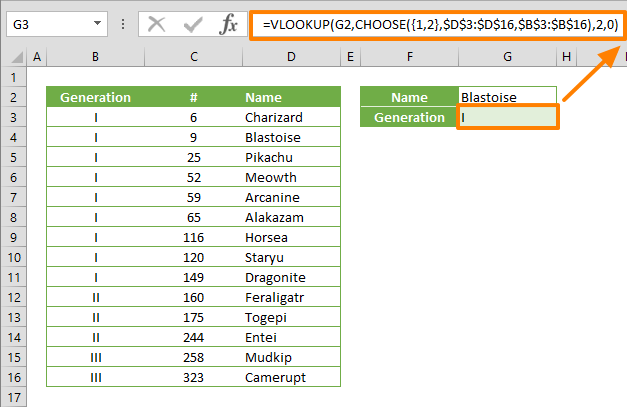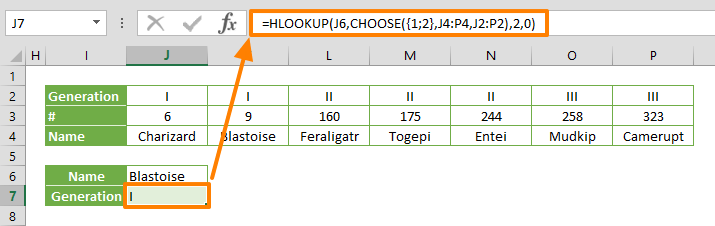Lookup functions are widely used in Excel models. However, one of the most popular lookup functions, VLOOKUP, can only search the left-most column in the search area. This function will fall short if you need to search in the middle of a table and return values from its left. In other words, if you want to search for a value in a range under column D, and return the matching value from column B, you will have to use a different method. This behavior is called a reverse lookup. In this guide, we are going to show you how to perform an Excel reverse lookup using the CHOOSE function.

## Returning an array using the CHOOSE function

The CHOOSE function can return an array, and we can swap the order of the columns afterwards. Let's take the same example above: The lookup range is under the column D, and the return range is under the column B. Using the CHOOSE function, we can tell the VLOOKUP that column D is the first (left-most) column and column B is the second column in an array.

The CHOOSE function can return an array if you enter an array or a reference as one of its value arguments. On the other hand, we need to return every value arguments of the outcome. To do this, we need to provide an array of index numbers, instead of a single value. For example; {1,2} makes the function return an array of value1 and value2 combined.

The following formula combines the arrays in D3:D16 and B3:B16 arguments, and returns an array incorporating both.

CHOOSE({1,2},D3:D16,B3:B16)

Now we are able to produce an array that fit our needs, the next step is to use the array in a lookup formula to perform reverse lookup.

## VLOOKUP and CHOOSE

You can use either the VLOOKUP and HLOOKUP functions in this method. In our example, we're going to be using VLOOKUP due to the fact that our ranges are vertical - e.g. D3:D13 and B3:B16.

Use the formula with CHOOSE as table_array of the VLOOKUP function. Since the array returned by the CHOOSE function only contains two columns, you will need to set 2 for col_index_num argument. This means that the column number between the two target columns are not relevant when performing a reverse lookup.

Let's establish a formula that can search for "Blastoise" in a range under column D, and and return the matching value under column B:

=VLOOKUP("Blastoise",CHOOSE({1,2},\$D\$3:\$D\$16,\$B\$3:\$B\$16),2,0)## HLOOKUP

If you want to use the CHOOSE function to provide an array for a HLOOKUP function, the two steps below are important:

1. Combine the horizontal ranges, e.g. K4:Q4 and K2:Q2
2. Provide a vertical array for the index_num argument, e.g. {1;2}

Regarding the second item, you need to use a semicolon (;) to separate 1 and 2 instead of a comma (,). While a comma indicates columns, a semicolon indicates rows.

=HLOOKUP(J7,CHOOSE({1;2},J5:P5,J3:P3),2,0)## Alternatives

You can also use the INDEX & MATCH combination, or the LOOKUP function to do an Excel reverse lookup. Please note that the LOOKUP function performs an approximate search, and assumes that your lookup array is sorted in ascending order. You can set the search for type by providing a [range_lookup] argument in VLOOKUP and HLOOKUP functions. You can also specify a search type when using MATCH function. As a result, the INDEX & MATCH combination is more viable alternative.